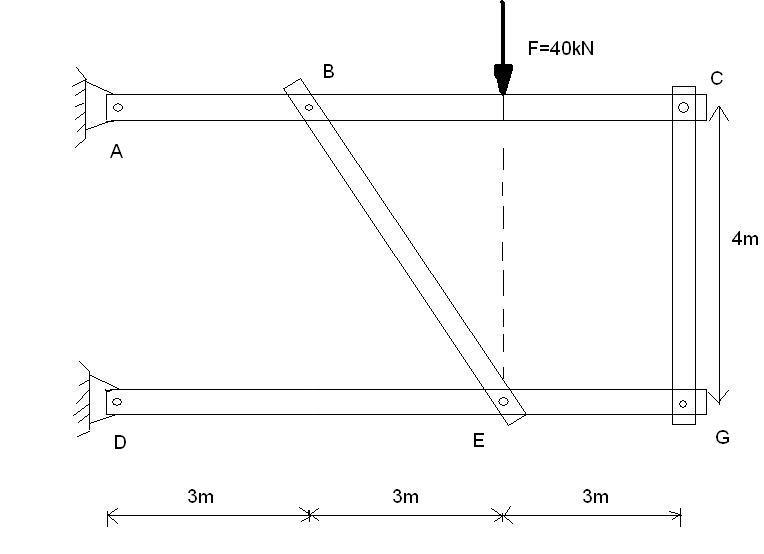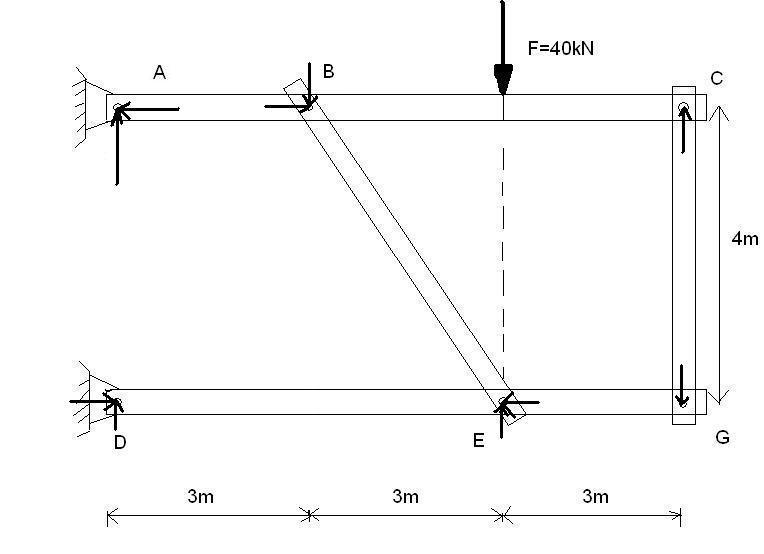# Help Needed: Solving Mechanics Problem in Norway

• eivert
In summary, this person is trying to figure out how to solve a mechanics problem, but is having difficulty because they do not have the right diagrams. They need to diagram each of the rods separately in order to solve the problem.f

#### eivert

Hello everyone

Iam practicing for my exam in mechanics and I can't figure out how to solve this problem. I need to find the forces in B and C.

Greetings from cold norwayThis is how I think the forces is working. Cant figure out if this is correct

the reactions at the supports look correct. you need to isolate the top portion of the structure (sum moments about C) to start getting answers.

When you attempt to solve the external reaction forces, you have 4 unknowns at those supports, but only the 3 equilibrium equations (sum of forces in x direction = 0, sum of forces in y direction = 0, and sum of moments about any point = 0). Thus, you'll need another equation to solve for all the support reactions. As haynewp has noted, you've got to start disassembling the frame with free body diagrams of the members in order to get the 4th equation you need, taking advantage in identifying the '2 force' members that will take axial load only.

I went ahead and solved it. There is actually one other step I used prior to summing moments about point C. You still have to isolate part of the structure as noted above which is the most important idea for this problem. I also found your vertical reaction at D looks like it is going in the wrong direction (should be pointing down). But I only knew this for sure after I went through the rest of the required process.

Welcome to PF!

Hi eivert! Welcome to PF from warm but rainy England!I'm sorry, but your diagram will not help.

(For example, on your diagram at B, there should be the force of BE on AC and the equal and opposite force of AC on BE)

You need separate diagrams for each of the rods.

Start with rods CG and BE (as PhanthomJay suggests).